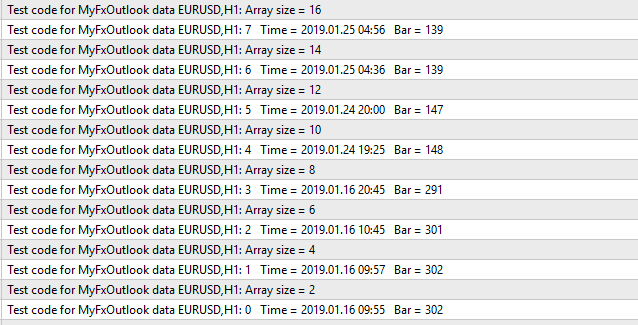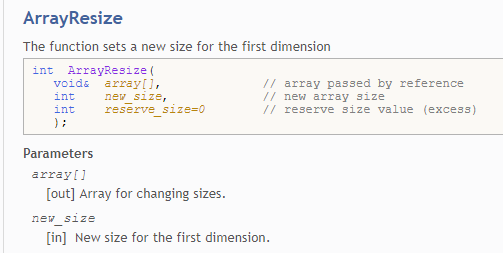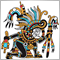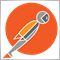# Array Resize????636

```struct data
{
datetime   open;
string     symb;
string     type;
double     lots;
double     price;
double     stoploss;
};

data arr[];
double bar_data[];

void OnStart()
{
int i = 0;
int num_data = 0;
ResetLastError();

if(file_handle!=INVALID_HANDLE)
{
while(!FileIsEnding(file_handle))
{
num_data++;
ArrayResize(arr,i+1,100);

if(arr[i].symb == ChartSymbol())
{
int bar = iBarShift(NULL,0,arr[i].open);
Print(i,"   Time = ",TimeToStr(arr[i].open) + "   Bar = ",bar);
ArrayResize(bar_data,i+1,100);
Print("Array size = ",ArraySize(bar_data));
bar_data[i] = bar;
bar_data[i] = arr[i].lots;
Sleep(200);
i++;
}
}
FileClose(file_handle);
}
else
{
PrintFormat("Failed to open file, Error code = %d",GetLastError());
}
}
//+------------------------------------------------------------------+
```Please can someone tell me why the array 'bar_data' increments by 2 every time? I thought 0 + 1 = 1 NOT 2!!1039

sd59:

Please can someone tell me why the array 'bar_data' increments by 2 every time? I thought 0 + 1 = 1 NOT 2!!

It's a multi-dimensional array. On resize by +1 it creates storage for 2 additional elements.636

I don't understand - this is MQL4 documentation - it should only be the first dimension that increments?1039

sd59:

I don't understand - this is MQL4 documentation - it should only be the first dimension that increments?

Yes. But ArraySize returns the number of all elements in your array. Try it:

```   double buffer[];
ArrayResize(buffer,1);
Print("size: ",ArraySize(buffer)); // --> 2
ArrayResize(buffer,3);
Print("size: ",ArraySize(buffer)); // --> 6
```34325

you need to use ArrayRange() instead of ArraySize()2392

You shouldn't use multi-dimensional arrays as a replacement for an array of objects/structs. You already use an array of structs so you could just make a filtered array of structs without changing the data-structure. Better yet you could simple rearrange your logic and encapsulate your methods and clean up your OnStart func.

```#property strict

#include <arrays/arrayobj.mqh>
#include <files/file.mqh>

class MyFxBookOrder : public CObject {
protected:
ENUM_TIMEFRAMES   m_barshift_tf;
int               m_shift;
public:
datetime       open_time;
string         symbol;
string         type;
double         lots;
double         price;
double         stoploss;
public:
MyFxBookOrder():m_barshift_tf(WRONG_VALUE),m_shift(-1){}
int            bar(ENUM_TIMEFRAMES tf=PERIOD_CURRENT);
};
int MyFxBookOrder::bar(ENUM_TIMEFRAMES tf=PERIOD_CURRENT) {
if (tf == m_barshift_tf && m_shift >= 0)
return m_shift;
m_shift = iBarShift(this.symbol, tf, this.open_time);
m_barshift_tf = tf;
return m_shift;
}

class MyFxBookOrderArray : public CArrayObj
{
public:
MyFxBookOrder  *operator[](int i) { return this.At(i); }
};
bool MyFxBookOrderArray::load_csv(string file_name, string filter_symbol=NULL) {
CFile f;
if (f.Open(file_name, FILE_READ|FILE_CSV, ',') == INVALID_HANDLE)
return false;
int file_handle = f.Handle();
while (!f.IsEnding()) {
MyFxBookOrder *order = new MyFxBookOrder();
if (filter_symbol == NULL || filter_symbol == order.symbol) {
return false;
}  else {
delete order;
}
}
return true;
}

void OnStart()
{
MyFxBookOrderArray orders;
for (int i=0; i<orders.Total(); i++) {
MyFxBookOrder *order = orders[i];
printf("index[%d], bar=%d, time=%s, lots=%.2f",
i, order.bar(), TimeToString(order.open_time), order.lots
);
}
}
}
//+------------------------------------------------------------------+```636

nicholi shen - thank you this is very nice but way above my programming level as you can see. I will need to do a lot of learning to understand your code.2392

sd59:

nicholi shen - thank you this is very nice but way above my programming level as you can see. I will need to do a lot of learning to understand your code.

Fair enough, but structs are just classes with all attributes made public. The reason you'll want to use classes in MQL instead of struct is because MQL doesn't allow you to use pointers to structs and that's the foundation of collections of dynamic objects in MQL. For your use you can simplify your specific code adding a bar atribute to your struct and then you can read the csv to a temp struct and if it matches your filter criteria then you copy to an array element. This isn't my preferred way to manage this type of data, but this should be more familiar to you.

```#property strict

struct MyFxbookOrder {
datetime   open_time;
string     symbol;
string     type;
double     lots;
double     price;
double     stoploss;
int        bar;
};

void OnStart()
{
int file_handle = FileOpen("Myfxbookdata.csv", FILE_READ|FILE_CSV, ',');
if (file_handle == INVALID_HANDLE)
return;

MyFxbookOrder filtered_orders[];

while(!FileIsEnding(file_handle)) {
MyFxbookOrder order;
if (order.symbol == _Symbol) {
order.bar = iBarShift(_Symbol, _Period, order.open_time);
int index = ArrayResize(filtered_orders, ArraySize(filtered_orders) + 1) - 1;
filtered_orders[index] = order;
}
}
FileClose(file_handle);
}```636

Thank you very much for taking time to do this.636

Hi nicholi shen,

I'm really sorry when I came to use your 'simplified' code I realised I still did not understand everything going on:

So I understand this is declaring filtered_orders[] as a structured array.

I do not understand what this is saying:

`MyFxbookOrder order;`

Or why I cannot simply print filtered_orders[index] as I get an error:

```'filtered_orders' - objects are passed by reference only        test 3.mq4      119     16
```

I will also need to sort the array (by bar number) - it looks like I will need to use Class structure for this - is this correct?

Unfortunately I try and read and understand but the 'English' is more like Martian to me! If you can point to documentation that can explain these things in simple English I'd be very grateful.2392

sd59:

Hi nicholi shen,

I'm really sorry when I came to use your 'simplified' code I realised I still did not understand everything going on:

So I understand this is declaring filtered_orders[] as a structured array.

I do not understand what this is saying:

Or why I cannot simply print filtered_orders[index] as I get an error:

I will also need to sort the array (by bar number) - it looks like I will need to use Class structure for this - is this correct?

Unfortunately I try and read and understand but the 'English' is more like Martian to me! If you can point to documentation that can explain these things in simple English I'd be very grateful.

I'd say at this point you really need to complete a tutorial on C++ fundamentals. Forgive my bluntness, but trying to hack together an understanding of how this stuff works by posting examples on this forum and waiting for an answer you cannot possibly understand is a total waste of your time.